Next: Eigenfunctions and Eigenvalues Up: Operators Previous: Basic Properties of Operators

### Linear Operators

Almost all operators encountered in quantum mechanics are linear operators. A linear operator is an operator which satisfies the following two conditions:
=
(43)
=
(44)

where c is a constant and f and g are functions. As an example, consider the operators d/dx and ()2. We can see that d/dx is a linear operator because
 (d/dx)[f(x) + g(x)] = (d/dx)f(x) + (d/dx)g(x) (45) (d/dx)[c f(x)] =
(46)

However, ()2 is not a linear operator because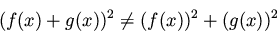(47)

The only other category of operators relevant to quantum mechanics is the set of antilinear operators, for which
(48)

Time-reversal operators are antilinear (cf. Merzbacher , section 16-11).Next: Eigenfunctions and Eigenvalues Up: Operators Previous: Basic Properties of Operators